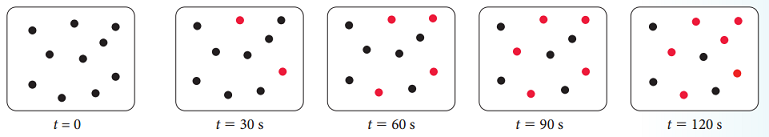# Problem: The images shown here depict the first-order reaction A → B at various times during the reaction process. The black circles represent reactant A and the red circles represent product B.What is the half-life of the reaction?

###### FREE Expert Solution

We’re being asked to determine the half-life of the reaction A → B based on the given diagram.

Recall that half-life is the time needed for the amount of a reactant to decrease by 50% or one-half

This means that we need to determine the time, t where the amount of A is reduced to one half.

We know that:

• reactant A is represented by black dots (•)
• product B is represented by red dots (•)

To do so, we need to count the number of black dots, A at t = 0, and determine t where:

• A = 1/2(Ainitial)
97% (374 ratings)###### Problem Details
The images shown here depict the first-order reaction A → B at various times during the reaction process. The black circles represent reactant A and the red circles represent product B.What is the half-life of the reaction?